BackReturn Home

FALLING BODIES THEORY
The Behavior Of Free Bodies In Space

Introduction

To understand the principles of unity it is imperative that the simple, basic tests which are outlined in the next few pages are completely understood. These were gravity tests, and were conducted at the site of an abandoned mine shaft in Northern Minnesota during the early 1960's. The shaft was approximately two-thousand feet in depth, and the impact plane was monitored by a high speed camera. In the tests where balls of unlike elements were dropped for comparison, equal weights were achieved by hollowing out the centers of those heavier elements, thus ensuring equal air restance factors.

Though our conclusions are founded on literally thousands of tests, we have here outlined only the most pivotal tests, all of which are simple enough to be reproduced by anyone who harbors doubts as to the accuracy of our results or the validity of our conclusions. To layman and physicist alike we would say: study these tests and understand the results, for in unity the actions of all falling bodies are the same – be that body a sub-atomic particle or a universe, and so by building a solid foundation through the study of these tests, the reader will find it simple to comprehend what the use of current nuclear technologies are doing to Earth, and why they must be abandoned if we are to save this planet.TEST ONE

Two iron balls of equal weight and size were placed six feet apart, and then were dropped simultaneously.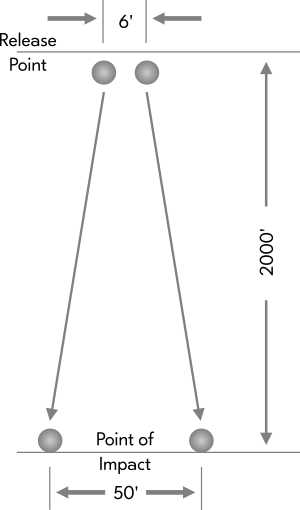Fig. 1

RESULT

The camera clearly indicated that the iron balls impacted at the same instant. Examination of the impact plane showed the balls were fifty feet apart when they impacted.

CONCLUSION

Identical elements of equal weight and size accelerate at exactly the same rate of speed, and repel one another if dropped as individuals - apart from each other.

TEST TWO

Four balls of equal weight and size, but composed of different elements were dropped simultaneously.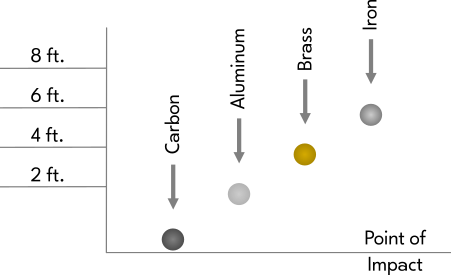Fig. 2

RESULT

Each different element accelerated at a different rate.

CONCLUSION

Different elements accelerate at different rates when falling through a given gravitational field.

TEST THREE

Twenty steel balls of equal weight and size were arranged in a pyramidal shape so that they were touching each other, and were then dropped simultaneously as a unit.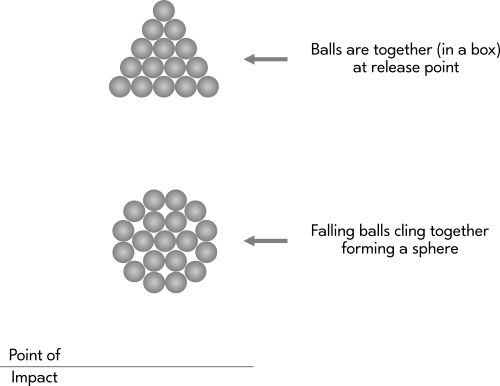Fig. 3

RESULT

The camera revealed that as the balls approached the impact plane they were still a unit, but had rearranged themselves from a pyramidal form to a spherical shape.

CONCLUSION

Individual bodies of like elements that fall in tight formation with other bodies will always cling together and will always arrange themselves into a spherical formation.

TEST FOUR

Three iron balls of equal weight and size were arranged as in figure 4, six feet apart from one another, and were then dropped simultaneously.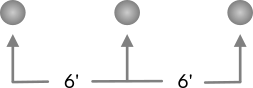Fig. 4

RESULT

The arrangement of the balls upon impact is diagrammed in figure 5.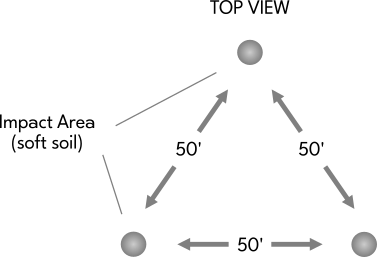Fig. 5

CONCLUSION

Bodies of like elements that fall through a given gravitational field as individuals drift away from each other at equal rates.

TEST FIVE

Twenty steel balls of equal weight and size were arranged and spaced one foot apart, as in figure 6. The balls were then dropped simultaneously.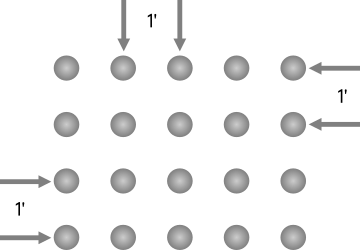Fig. 6

RESULT

Figure 7 shows the arrangement of the balls an instant before imapct as revealed by the camera.

[insert image]
Fig. 7

Figure 8 shows the placement of the balls from the top view after impact.

[insert image]
Fig. 8

CONCLUSION

Individual bodies that fall as a mass in loose formation drift apart on a 360° horizontal plane and on an 180° vertical plane simultaneously.

TEST SIX

Two iron balls of equal weight and size were painted around the circumference as in figure 9. They were then placed in the drop box so that the lines were exactly horizontal, and then were dropped simultaneously.

[insert image]
Fig. 9

RESULT

An instant before impact the camera showed that the painted lines were no longer in their original position. After impact the two balls rolled away in the same direction.

CONCLUSION

All bodies will develop a given rotation. This is true whether one speaks of a single body, a mass of bodies in loose formation, or a mass of bodies in close formation. These rotating bodies, if dropped as individuals (apart from each other) repel one another at equal rates.

TEST SEVEN

Iron balls of equal weight and size were suspended on six foot arms and were rotated in a clockwise direction, horizontally, around a pivot point. An antenna was placed in close tolerance to the circumference of the rotating balls. Attached to the antenna were extremely sensitive detection devices, which were in turn connected to an oscilloscope.

[insert image]
Fig. 10

RESULT

The oscilloscope display showed a donut shaped form which appeared at the instant a ball passed the antenna as shown in figure 11. The oscilloscope appeared to not retain any stigmentation.

[insert image]
Fig. 11

CONCLUSION

A body in motion produces a field and/or force which appears donut shaped on an oscilloscope display. This field (and/or force) has not been identified by the scientific community to date.

TEST EIGHT

An identical apparatus to that used in test seven was constructed. The two units were placed parallel to each other and were timed so that as the arms rotated, the balls would pass within ¼ inch of each other at point x.

[insert image]
Fig. 12

RESULT

Each time two balls met and passed each other at point x, a tremendous thump was heard, and the test stand (a six inch diameter shaft mounted in concrete) reacted violently.

As the balls approached each other in their rotation the oscilloscope showed donut shapes as in test seven. At the instant the balls were closest to each other in their rotation the oscilloscope showed sine waves. As the balls again began to move away from each other in their rotation, the donut shapes reappeared on the oscilloscope display, as shown in figure 13.

[insert image]
Fig. 13

CONCLUSION

The field (and/or force) which surrounds a body in motion repels the field (and/or force) which surrounds a second body in motion. The field (and/or force) that surrounds a body in motion produces an energy which is not polarized. It appears as a bubble (a donut, or a circle) on an oscilloscope display. When this field (and/or force) is forced into collision with the field (and/or force) that surrounds a second body, the bubbles break, then unite with each other to produce polarized energy, i.e.: sine waves.

NOTE:

Test eight was repeated with many variations in an effort to isolate the source of the "thump". It was determined that an increase in the distance between the two balls at point x would result in a decrease in the intensity of the thump. Even in a test where the balls came no closer to each other than six inches, however, the thump continued to be a notable factor.

In other variations of this basic test, many different R.P.M.'s were employed. It was found that the thumping increased progressively as the R.P.M. was increased. It was then discovered that the intensity of the thumping became progressive at the rate of velocity squared, and that with an increase of the distance between the balls at point x the thumping decreased at the rate of distance squared. Though these findings may seem irrelevant to the reader at the present time, they take on infinite importance in allowing one to understand the actions of larger, celestial falling bodies.

COMMENT

Galileo was correct in his conclusion that iron balls accelerate at the same rate of speed within a given gravitational field, however, his work was grossly incomplete in that he'd never considered the acceleration of any element in respect to iron. Issac Newton then placed his mathematical calculations on the law of Galileo which stated that all falling bodies accelerate at the same rate of speed, and so developed a law which stated that gravity was always an attracting force. Albert Einstein then based his theories of relativity and general relativity on the work of Newton. Today we observe the entire scientific community basing their work on Einstein. Any thinking person can quickly see the danger in such a situation as our modern scientists plunge recklessly forward into technologies based on incomplete experiments and faulty theory. In absolute sincerity we say: they know not what they do.